# Getting started

In the following sections we'll look at how to get started using Popper for writing and running tests.

Popper consists of two parts:

• A library `popper` — containing functions for building and executing tests.

• A library `ppx_deriving_popper` — for deriving functions to sample and compare test data for custom data-types.

## Installation

Installing `ppx_deriving_popper` via OPAM brings in both the ppx and the testing library.

``````> opam install ppx_deriving_popper
``````

Similarly, if you're using the ppx, adding a preprocessor dependency on `ppx_deriving_popper` includes the runtime library `popper` as well.

Currently, using the `[@@deriving ... popper]` attribute also requires dependencies for deriving `show` and `ord` for your data types. Below is an example dune file for defining a test. It assumes a file `run.ml`:

``````(test
(name run)
(preprocess
(pps ppx_deriving.show ppx_deriving.ord ppx_deriving_popper)))
``````

## Unit tests

As a first example, here is a simple unit-test verifying that the function `List.rev` behaves as expected when applied with the list `[1; 2; 3]`:

``````open Popper

let () =
check @@ fun () ->
equal Comparator.(list int) (List.rev [ 1; 2; 3 ]) [ 3; 2; 1 ]
``````

When run with `dune runtest`, it produces the following output: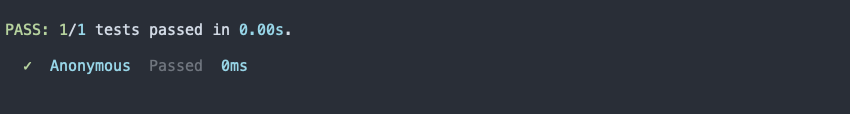The function `check` is for running a single (anonymous) test. Its signature is:

``````val check : ?⁠config:Config.t -> (unit -> Proposition.t Sample.t) -> unit
``````

It takes a function that produces a value of type `Proposition.t Sample.t` and executes it.

We'll discuss the `Sample.t` part in the next sections. The `Proposition.t` value is the result of the test, and evaluates to one of the following values:

• `pass` — the proposition is `true`, the test passes.
• `fail` — the proposition is `false`, the test fails.
• `discarded` — the proposition is neither `true` nor `false`, the test is discarded.

The function `equal` constructs a proposition stating that two values are equal according to the given `Comparator.t` value:

``````val equal : ?⁠loc:string -> 'a Comparator.t -> 'a -> 'a -> Proposition.t Sample.t
``````

A value of type `a Comparator.t` wraps a compare function and a pretty-printer for the type `a`. For convenience, the function returns in the `Sample.t` context.

In this case we construct an `int list Comparator.t` value using the `list` combinator from the `Comparator` module.

Let's see what happens when a test fails. Modifying the example above slightly:

``````let () =
check @@ fun () ->
equal Comparator.(list int) (List.rev [ 1; 2; 3 ]) [ 2; 3; 1 ]
``````

This yields: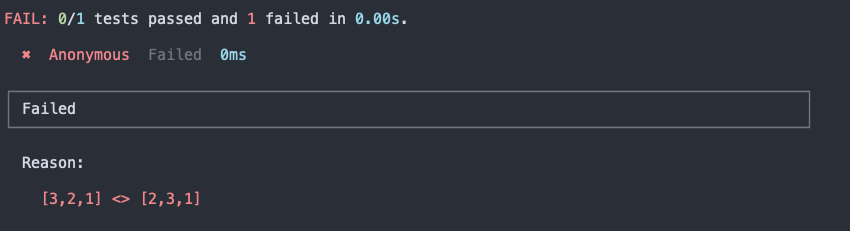The comparator embeds a pretty-printer for being able to explain why a particular proposition failed for the given arguments.

## Property-based tests

A property-based test is one that, instead of verifying a concrete test-case, confirms that a certain property holds for a large number of sampled data points. The technique was popularized by the Haskell library QuickCheck. Using property-based testing effectively is a topic of its own and is independent of the particular choice of library or language. Here is a good article with examples using F#.

The school-book example for a property-based test is one that verifies that reversing a list twice gives the same list back. Using Popper, it can be expressed as follows:

``````open Popper
open Sample.Syntax

let () =
check @@ fun () ->
let* xs = Sample.(list int) in
equal Comparator.(list int) (List.rev (List.rev xs)) xs
``````

In the test, `xs` is a sample of type list of integers. The test returns a proposition that asserts that after reversing `xs` twice we end up with the same list.

The `let*` syntax defined in the `Sample.Syntax` module provides a convenient method for expressing this in a declarative way.

When run, it produces the following output: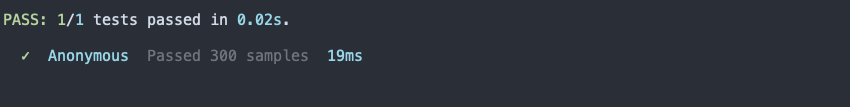The function `check` evaluates the property multiple times (300 by default), by drawing different samples and verifying the resulting proposition for each of them.

Note

In Popper, there is no fundamental difference between unit tests and property-based tests. They are constructed and run using the same functions.

To see what happens when a test fails, here is an example to confirm that `List.sort` returns a sorted list, but where the condition does not account for lists containing duplicated elements.

``````let rec is_sorted = function
| x :: y :: ys -> x < y && is_sorted (y :: ys)
| _ -> true

let () =
check @@ fun () ->
let* xs = Sample.(list int) in
is_true (is_sorted @@ List.sort Int.compare xs)
``````

The function `is_true` is used to construct a proposition stating that the given boolean condition is `true`.

This test fails with: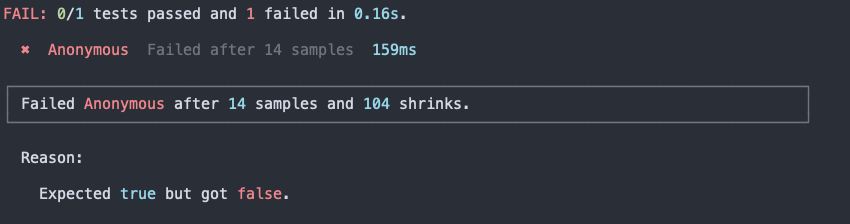The output is not very informative as all it says is that the proposition failed because the boolean condition was `false`. In order to actually observe the drawn sample for which the condition `is_sorted` did not hold, we may add add some logging. The simplest way is to use the function `Sample.with_log`:

``````val with_log
: string
-> (Stdlib.Format.formatter -> 'a -> unit)
-> 'a Sample.t
-> 'a Sample.t
``````

It takes a string value for the key, a formatter, and a sampler. It returns a new `Sample.t` value that also records the sampled values.

To quickly obtain a formatter (aka pretty-printer) for lists of integers, you may derive it using `[@@deriving show]`. We'll soon look at how to also derive samplers and comparators as well. For now:

``````type int_list = int list [@@deriving show]

let () =
check @@ fun () ->
let* xs = Sample.(with_log "xs" pp_int_list (list int)) in
is_true (is_sorted @@ List.sort Int.compare xs)
``````

The result, when run: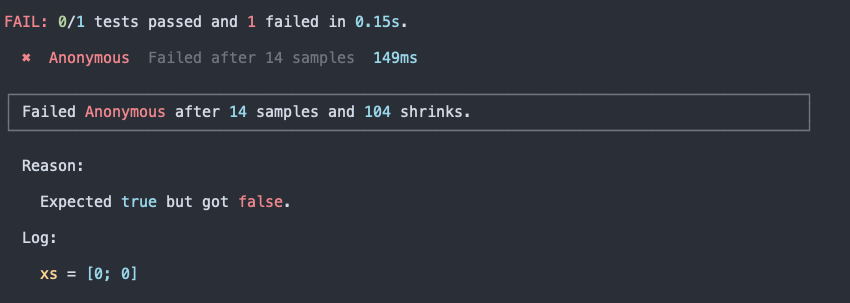The log section now displays the sampled value `xs` for which the test failed.

A property-based test such as the one above is run multiple times, a simple unit-test is only run once. How does `check` know how to distinguish between the two? The answer is that a simple unit test does not consume any input when generating the resulting proposition. Any property-based test must consume some data for drawing (pseudo-random) samples. A value of type `a Sample.t` is really a parser that reads input from a stream of numbers (`int32` values) and produces a result of type `a`. By varying the input stream we feed to the parser, we obtain different results.

Note

Whenever a failing sample is encountered, the `check/run` function attempts to find a smaller counter-example by shrinking the input stream that was used for producing the sample.

## Test suites

The function `check` is convenient for quickly running individual tests, but when working with multiple tests it's likely better to bundle and run them together.

There are three essential functions for this purpose:

• `test` for creating a test.
• `suite` for naming and grouping tests.
• `run` for running a test (or a test suite).

Their signatures are:

``````val test : ?⁠config:Config.t -> (unit -> Proposition.t Sample.t) -> Test.t
val suite : (string * Test.t) list -> Test.t
val run : ?⁠config:Config.t -> Test.t -> unit
``````

Here's how you can combine the tests from above in a suite, and run it:

``````let test_rev =
test @@ fun () ->
equal Comparator.(list int) (List.rev [ 1; 2; 3 ]) [ 3; 2; 1 ]

let test_rev_twice =
test @@ fun () ->
let* xs = Sample.(list int) in
equal Comparator.(list int) (List.rev (List.rev xs)) xs

let test_sort =
test @@ fun () ->
let* xs = Sample.(with_log "xs" pp_int_list (list int)) in
is_true (is_sorted @@ List.sort Int.compare xs)

let tests =
suite
[ ("Reverse", test_rev)
; ("Reverse twice", test_rev_twice)
; ("Sort", test_sort)
]

let () = run tests
``````

Hopefully the definitions are straight-forward. There are three tests which are defined using the function `test`. They are packed together by the `suite` combinator and executed with `run`.

This results in the output: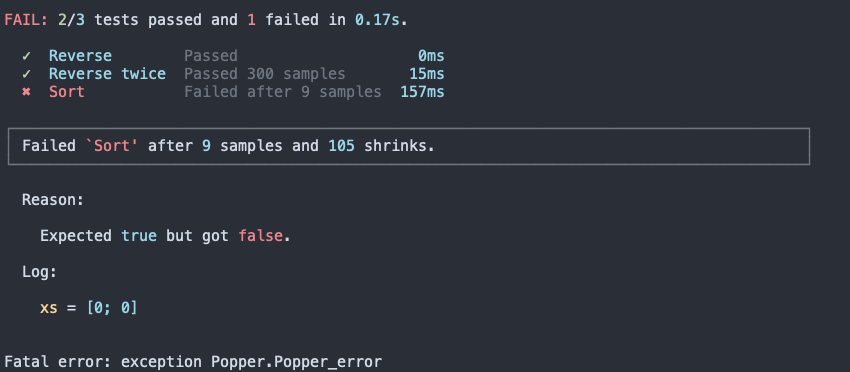Note that the value `tests` has the same type as `test_rev`, `test_rev_twice`, and `test_sort`, namely `Test.t`. That means that test suites may also be nested. For instance, as in:

``````let rev_suite =
suite [ ("Simple list", test_rev); ("Reverse twice", test_rev_twice) ]

let tests = suite [ ("Reverse", rev_suite); ("Sort", test_sort) ]

let () = run tests
``````

Which yields: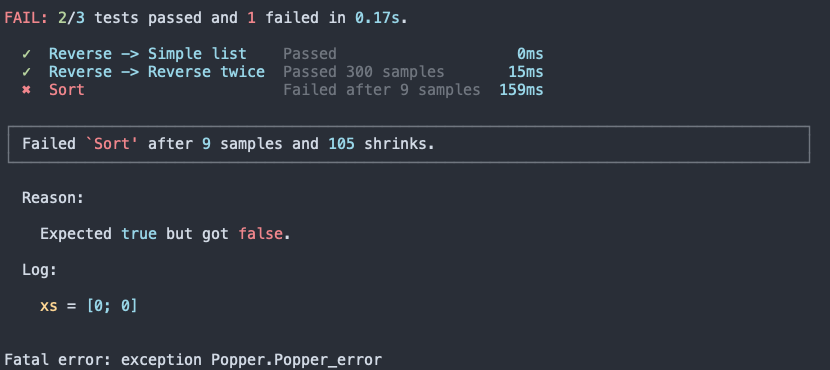The nesting is reflected in the output, such as `Reverse -> Simple list`.

Note

There is only one type of tests — `Test.t` values — and tests may be nested arbitrarily using the `suite` combinator.

## Deriving samples

For property-based tests, we sample data of some type and use the values for verifying certain properties. There are two options for sampling the input data:

1. Sample the data and define comparators manually (as done in the examples above).
2. Derive sample and comparator functions using the `ppx_deriving_popper` ppx.

For example, consider a simple data type, `exp`, for representing boolean expressions:

``````type exp =
| Lit of bool
| And of exp * exp
| Or of exp * exp
| Not of exp
``````

Along with an evaluation function, `eval`, with a signature:

``````val eval : exp -> bool
``````

What if we would like to write a test that verifies that `Lit false` is the identity expression for `Or`? That is, for any expression `e`, the following proposition should evaluate to true/pass:

``````eval e = eval (Or (Lit false, e))
``````

How can we sample `exp` values? We'll cover how to do this manually in a bit but the easiest way forward is to derive it. All that is needed is adding an attribute `[@@deriving .. popper]`. It also requires deriving `ord` and `show` for the comparator part.

``````type exp =
| Lit of bool
| And of exp * exp
| Or of exp * exp
| Not of exp
[@@deriving show, ord, popper]
``````

At the preprocessing stage, the popper ppx generates two functions:

``````val exp_comparator : exp Comparator.t
val exp_sample : exp Sample.t
``````

Alternatively, if you only care about deriving the sample function you may skip `show` and `ord` and use `sample` instead of `popper`, i.e.:

``````type exp = ... [@@deriving sample]
``````

Before writing the property-based test, here's (a buggy) implementation of `eval`:

``````let rec eval = function
| Lit b -> b
| And (e1, e2) -> eval e1 && eval e2
| Or (e1, e2) -> eval e1 && eval e2
| Not b -> not @@ eval b
``````

Using the `exp_sample` function, the test is straight forward:

``````let test_or =
test @@ fun () ->
let* e = exp_sample in
is_true (eval e = eval (Or (Lit false, e)))

let () = run test_or
``````

The test now fails after 5 successful samples: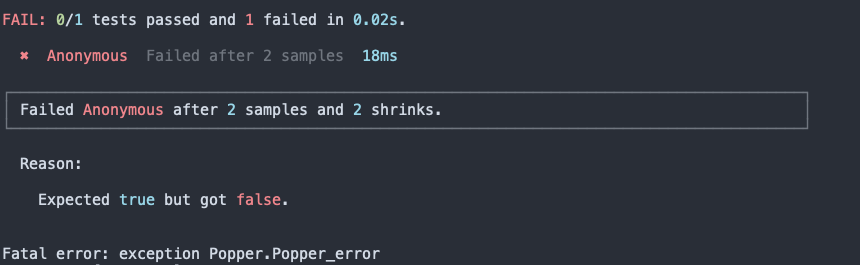Again, in order to actually see the expression that was generated in order for the proposition to fail, we need to add some logging:

``````let test_or =
test @@ fun () ->
let* e = Sample.with_log "e" pp_exp exp_sample in
is_true (eval e = eval (Or(Lit false, e)))

let () = run test_or
``````

The `with_log` requires providing a pretty-printer. We use `pp_exp` which is derived by `show`.

When running the test, you now also see the logged values in the output: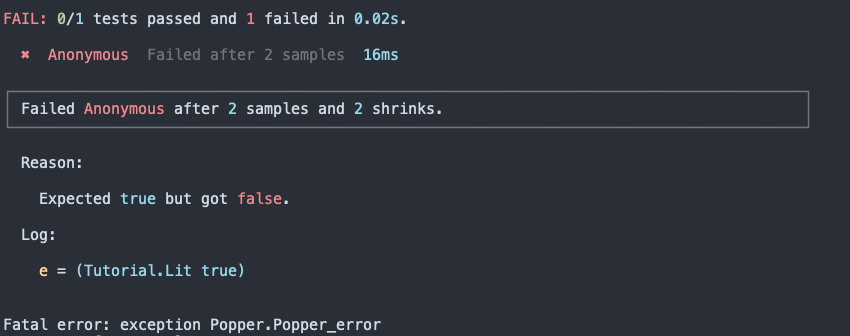Note

Deriving popper for a type called `t`, generates functions called `sample` and `comparator`, rather than `t_sample` and `t_comparator`. This is to mirror the deriving behavior for `show` and `ord`.

## Combining propositions

Just like tests can be composed into test suites, propositions can also be bundled. There are two functions:

``````val all : Proposition.t Sample.t list -> Proposition.t Sample.t

val any : Proposition.t Sample.t list -> Proposition.t Sample.t
``````

They both take a list of propositions (for convenience ```Proposition.t Sample.t``` values) and collapse them into a single proposition. As the names suggest, `all` requires all propositions to pass, and `any` requires at least one to do so, in order to return a successful result.

Here's an example:

``````let test_id =
test @@ fun () ->
let* e = exp_sample in
all
[ is_true (eval e = eval (Or (Lit false, e)))
; is_true (eval e = eval (And (Lit true, e)))
]
``````

You may also use the pure combinators from the Proposition module, which do not return in the `Sample` context.

## Configurations

As you may have noticed, the functions `test`, `check`, and `run` all take an optional configuration argument called `config`. This allows overriding some settings. Either at the level of an individual test or for all tests when passed to `run`. In case there are overlapping settings, the ones defined at the lowest level, i.e. the ones passed to an individual `test` take precedence.

Here are some examples of what may be configured:

• `num_samples` — for specifying the number of samples to be drawn for property-based tests. The default value is 300.
• `verbose` — whether or not the logged values should be displayed for each sample.
• `max_size` — this is a parameter that controls the maximum size parameter for data-structures like lists. It defaults to 100.

For the complete list, see the module Popper.Config.

First, let's see what happens when we run a test configured with `verbose`. Here's a modified version of the `test_or` function from above:

``````let test_or =
test ~config:Config.verbose @@ fun () ->
let* e = Sample.with_log "e" pp_exp exp_sample in
is_true (eval e = eval (Or(Lit false, e)))

let () = run test_or
``````

You now see what all the drawn expression samples look like: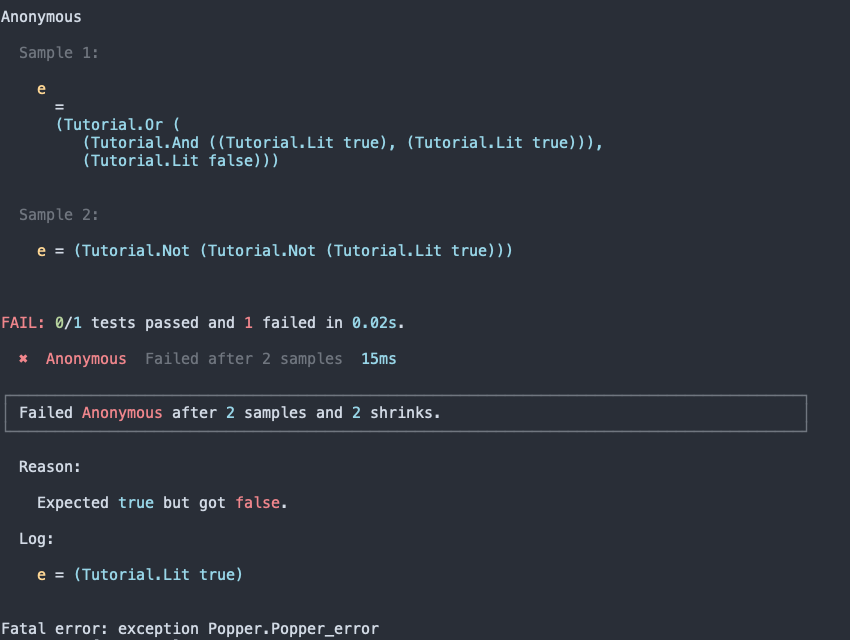Also changing the number of samples to be tested can be done by combining `Config.t` values with the `Config.all` combinator. For example:

``````let test_not =
let config = Config.(all [verbose; num_samples 1000]) in
test ~config @@ fun () ->
let* e = Sample.with_log "e" pp_exp exp_sample in
is_true (not (eval e) = eval (Not e))

let () = run test_not
``````

The output confirms that the number of evaluated samples is now 1000: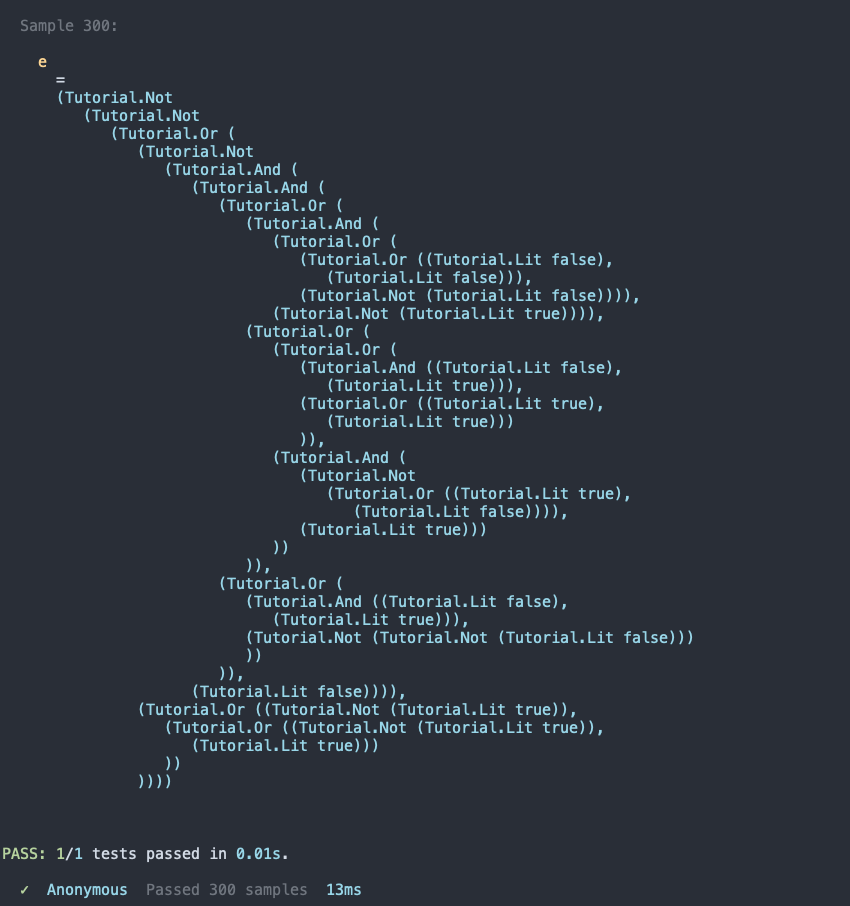Note

If property-based tests are slow to execute, you may decrease the number of samples using `Config.num_samples` or decrease the max-size parameter with `Config.max_size`. This, of course, limits the test coverage.

## Custom samples

It is not always feasible to derive generic sample functions for the data-types you need for testing. Specifically when:

1. The data-type is abstract.
2. There are invariants that the samples must satisfy.

In those cases you need to write your own sample function.

To give an example, consider testing an module, `Image`, with the following signature:

``````type color = White | Black | Transparent
type t
val make : width:int -> height:int -> (x:int -> y:int -> color) -> t
val render : t -> string
val invert : t -> t
``````

Here's an implementation:

``````module Image = struct
type color = White | Black | Transparent
type t =
{ width : int
; height : int
; get_pixel : x:int -> y:int -> color
}

let make ~width ~height get_pixel = { width; height; get_pixel }

let render { width; height; get_pixel } =
List.init height (fun y ->
List.init width (fun x ->
match get_pixel ~x ~y with
| White -> "w "
| Black -> "b "
| Transparent -> "- ")
|> String.concat "")
|> String.concat "\n"

let invert { width; height; get_pixel } =
let get_pixel ~x ~y =
match get_pixel ~x ~y with
| White -> Black
| Black -> White
| Transparent -> White
in
{ width; height; get_pixel }
end
``````

Now say you would like to write a test for a property that states that inverting the image twice results in the same image when rendered. However, it is not possible to derive a sample function for the abstract type `Image.t`. Further, even if the type had been exposed, we need to constrain the `width` and the `height` parameters. Luckily, writing the sample function yourself is straight forward — it's just a matter of generating the different parameters. Here is a version where the width and height varies between `0` and `14`:

``````let sample_img =
let* width = Sample.Int.range 0 15 in
let* height = Sample.Int.range 0 15 in
let* lookup =
Sample.fn
(Sample.one_value_of [ Image.Black; Image.White; Image.Transparent ])
in
let get_pixel ~x ~y = lookup (x, y) in
Sample.return (Image.make ~width ~height get_pixel)
``````

And here is how to use the function for defining a test:

``````let test_invert_twice =
test @@ fun () ->
let* img = sample_img in
equal
Comparator.string
(Image.render img)
(Image.render (Image.invert (Image.invert img)))

let suite = suite [ ("Invert twice", test_invert_twice) ]
let () = run suite
``````

Running the test reveals a bug: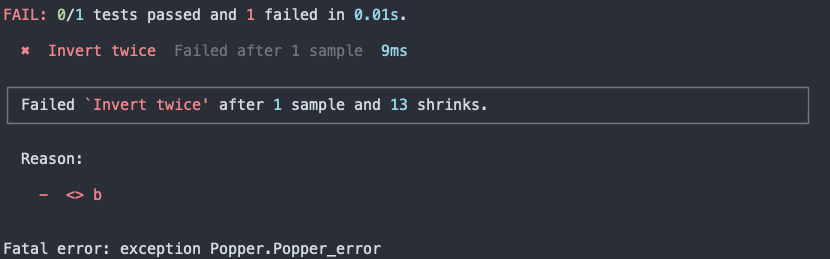The test failed after the first sample, and 13 successful shrink-steps were performed.

Note

An important feature of Popper is that shrinking is embedded. It means that when a failing sample is encountered and an attempt is made to shrink it, the invariants used for constructing the sample are always respected! Thus, in the example above — with the `Image.t sample` — shrinking would never cause, say, the height to be negative.

You can read more about the mechanisms behind sampling and shrinking in the how it works section.